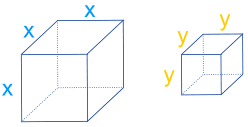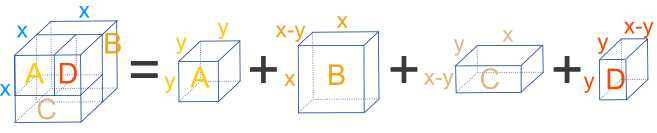# Difference of Two Cubes

There is a special case when multiplying polynomials that produces this: a3 − b3

## Polynomials

A polynomial looks like this:example of a polynomial

## Difference of Two Cubes

The Difference of Two Cubes is a special case of multiplying polynomials :

(a−b)(a2+ab+b2) = a3 − b3

It comes up sometimes when solving things, so is worth remembering.

And this is why it works out so simply (press play):

## Example from Geometry:

Take two cubes of lengths x and y:The larger "x" cube can be split into four smaller boxes (cuboids), with box A being a cube of size "y":The volumes of these boxes are:

• A = y3
• B = x2(x − y)
• C = xy(x − y)
• D = y2(x − y)

But together, A, B, C and D make up the larger cube that has volume x3:

 x3 = y3 + x2(x − y) + xy(x − y) + y2(x − y) x3 − y3 = x2(x − y) + xy(x − y) + y2(x − y) x3 − y3 = (x − y)(x2 + xy + y2)

Hey! We ended up with the same formula! Thank goodness.

## Sum of Two Cubes

There is also the "Sum of Two Cubes"

By changing the sign of b in each case we get:

(a+b)(a2−ab+b2) = a3 + b3

(note the minus in front of "ab" also)